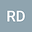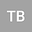Shell equations in terms of Günter’s derivatives, derived by the Г-convergence
••Tengiz BUCHUKURI
Tbilisi State University, A. Razmadze Mathematical Institute
Author Profile## Abstract

A mixed boundary value problem for the L\’ame equation in a thin layer $\Omega^h:\cC\times[-h,h]$ around a surface $\cC$ with the Lipshitz boundary is investigated. The main goal is to find out what happens when the thickness of the layer tends to zero $h\to0$. To this end we reformulate BVP into an equivalent variational problem and prove that the energy functional has the $\Gamma$-limit being the energy functional on the mid-surface $\cC$. The corresponding BVP on $\cC$, considered as the $\Gamma$-limit of the initial BVP, is written in terms of G\”unter’s tangential derivatives on $\cC$ and represents a new form of the shell equation. It is shown that the Neumann boundary condition from the initial BVP on the upper and lower surfaces transforms into a right-hand side term of the basic equation of the limit BVP.
25 Feb 2020Submitted to Mathematical Methods in the Applied Sciences
01 Mar 2020Submission Checks Completed
01 Mar 2020Assigned to Editor
01 Mar 2020Reviewer(s) Assigned
27 Nov 2020Review(s) Completed, Editorial Evaluation Pending
28 Nov 2020Editorial Decision: Revise Minor